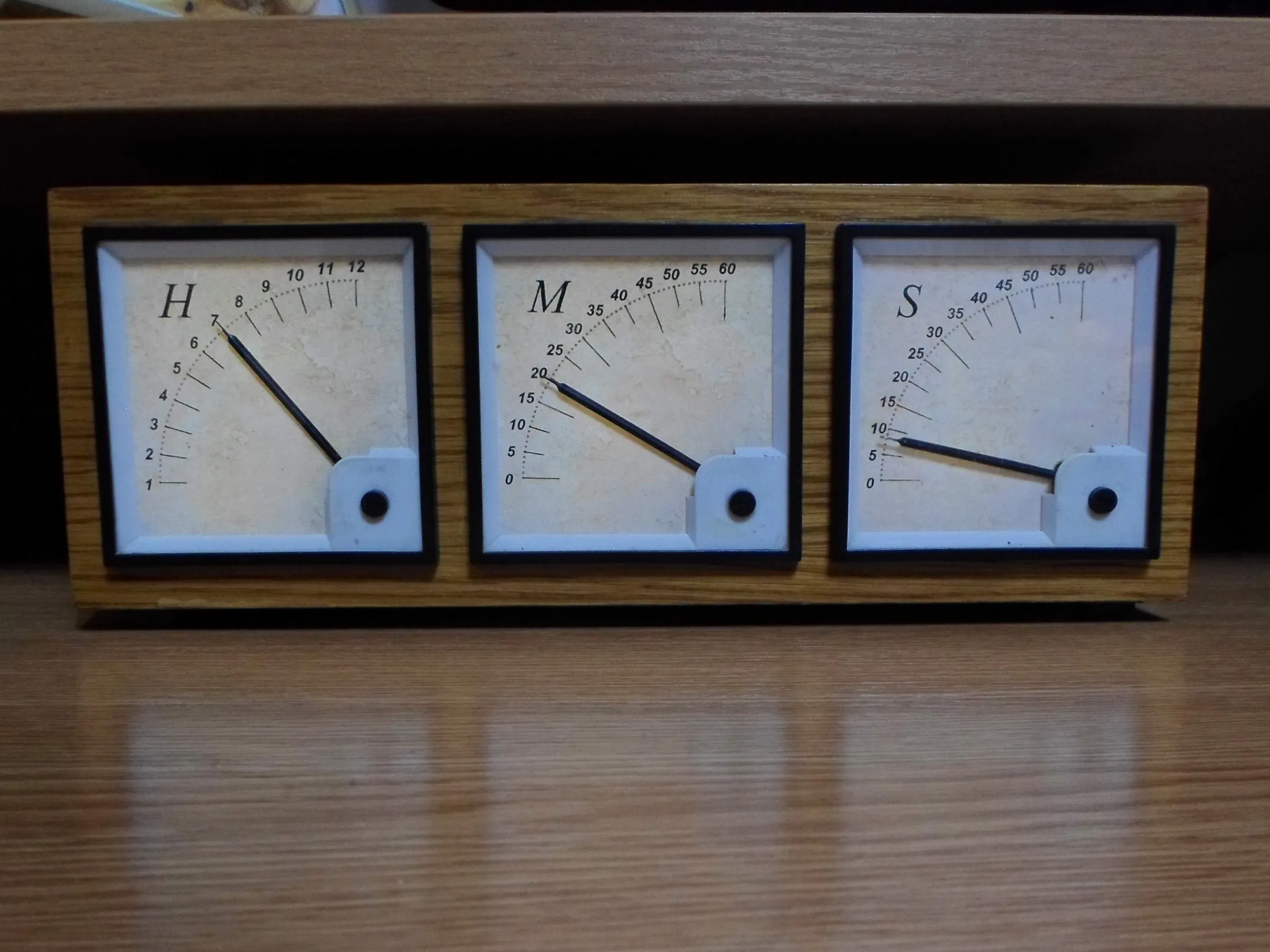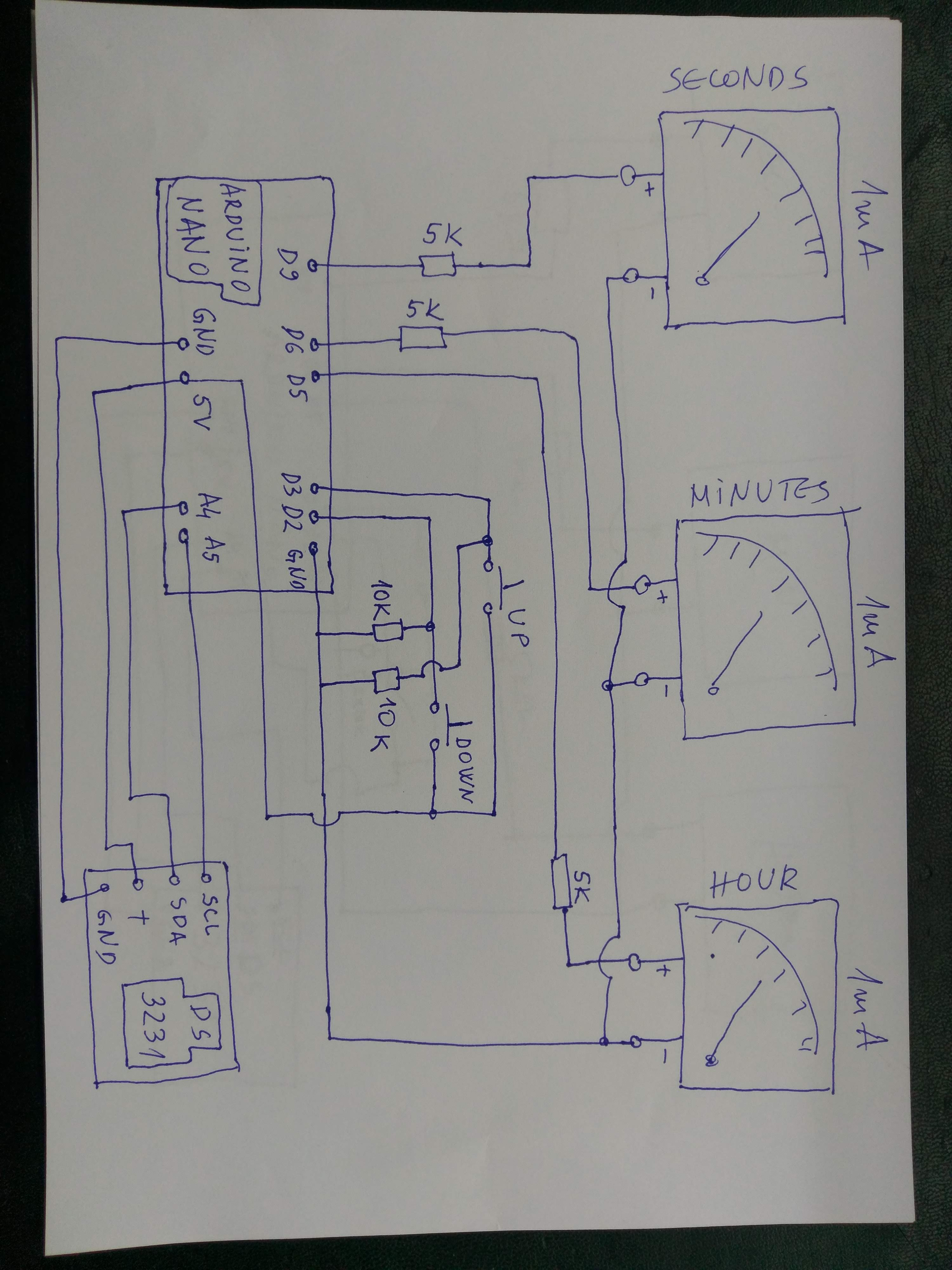Project tutorial# Arduino Analog Panel Meter Clock © GPL3+

Nice looking wooden analog style clock with Arduino Nano and 1mA ammeters.

• 10,709 views
• 65 respects

## Apps and online services

I built this nice-looking wooden analog style clock with an Arduino Nano and 1mA ammeters. I got an idea from here. For displaying the seconds, a part of the code has been added. Also added is a part that, when running, passes through the entire scale from zero to max. value for calibrating purposes. Depending on what ampermeters we use, the value of the 5k resistors can be different. Because all meters are slightly different, the PWM values need to be adjusted based on your design. You will have to change some values because it is set to work specifically with my meters.

## Code

##### codeArduino
```/*
Analog Panel Meter - Clock ONLY Mode

Credits:
bildr - DS1307 RTC code http://bildr.org/2011/03/ds1307-arduino/
_____________________
Wiring goes as follows:

- USB red wire (5v) to VCC
- USB black wire to GND

- Buttons - Left/Down to Digital 2  (follow arduino tutorial: http://arduino.cc/en/uploads/Tutorial/button.png )
- Right/Up to Digital 3

- DS1307 RTC  - SDA to Analog 4
- SCL to Analog 5

- Left Analog Meter to Digital 5 (PWM)
- Right Analog Meter to Digital 6 (PWM)
_-Seconds Analog meter to Digital 9_(PWM)_____________________

Note:
Because all meters are slightly different, the PWM values
need to be adjusted based on your design. Feel free to use
my code, but you will have to change some values because
it is set to work specifically with my meters.

*/

#include "Wire.h"
byte zero = 0x00; //workaround for issue #527

int downButton = 2;    // down push button to adjust time (pin 2)
int upButton = 3;      // up push button to adjust time (pin 3)

int hours = 0;
int minutes = 0;
int seconds = 0;
int lastMinute = 0;
int lastSecond = 0;
int leftMeter = 5;       // left meter (hours) is attached to pin 5
int rightMeter = 6;     // right meter (min) is attached to pin 6
int secMeter=9;         //seconds meter (sec)is attached to pin 9
void setup(){
Wire.begin();
Serial.begin(9600); //view time in serial monitor

pinMode(leftMeter, OUTPUT);      // Initialize Outputs & Inputs
pinMode(rightMeter, OUTPUT);
pinMode(secMeter, OUTPUT);

digitalWrite(leftMeter, HIGH);   // Test meters on startup
digitalWrite(rightMeter, HIGH);
digitalWrite(secMeter, HIGH);
digitalWrite(13, HIGH);
delay (1000);
digitalWrite(leftMeter, LOW);
digitalWrite(rightMeter, LOW);
digitalWrite(secMeter, LOW);
digitalWrite(13, LOW);
delay(1000);
}

void loop(){
printDate();
delay(75);

// Changing the time with buttons
byte second = seconds; // Don't get confused: second is not the same as seconds
byte minute = minutes; // Don't get confused: minute is not the same as minutes
byte hour = hours;     // Don't get confused: hour is not the same as hours
if (minutes > 0){
--minute;
}else{
minute = 59;
if (hours > 0){
-- hour;
}else{
hour = 23;
}}
Wire.write(zero); //stop Oscillator

Wire.write(decToBcd(second));
Wire.write(decToBcd(minute));
Wire.write(decToBcd(hour));

Wire.write(zero); //start

Wire.endTransmission();
}

byte second = 0;
byte minute = minutes; // Don't get confused: minute is not the same as minutes
byte hour = hours;     // Don't get confused: hour is not the same as hours
if (minutes < 59){
++minute;
}else{
minute = 0;
if (hours < 23){
++ hour;
}else{
hour = 0;
}}
Wire.write(zero); //stop Oscillator

Wire.write(decToBcd(second));
Wire.write(decToBcd(minute));
Wire.write(decToBcd(hour));

Wire.write(zero); //start

Wire.endTransmission();
}
}

byte decToBcd(byte val){
// Convert normal decimal numbers to binary coded decimal
return ( (val/10*16) + (val%10) );
}

byte bcdToDec(byte val)  {
// Convert binary coded decimal to normal decimal numbers
return ( (val/16*10) + (val%16) );
}

void printDate(){

// Reset the register pointer
Wire.write(zero);
Wire.endTransmission();

hours = bcdToDec(Wire.read() & 0b111111); //24 hour time

//print the date
Serial.print(hours);     // Un-comment to view time in serial monitor
Serial.print(":");       //
Serial.println(minutes); // Don't forget to un-comment 'Serial.begin' in 'void setup' above

// Specific meter levels for each hour
// You will likly have to adjust these values.
// Play around with the values until you get what you want.

if (lastMinute != minutes){
if (hours == 1 || hours == 13){
analogWrite(leftMeter, 0); // adjust left (hour) meter level
}
if (hours == 2 || hours == 14){
analogWrite(leftMeter, 29); // adjust left (hour) meter level
}
if (hours == 3 || hours == 15){
analogWrite(leftMeter, 52); // adjust left (hour) meter level
}
if (hours == 4 || hours == 16){
analogWrite(leftMeter, 75); // adjust left (hour) meter level
}
if (hours == 5 || hours == 17){
analogWrite(leftMeter, 98); // adjust left (hour) meter level
}
if (hours == 6 || hours == 18){
analogWrite(leftMeter, 130); // adjust left (hour) meter level
}
if (hours == 7 || hours == 19){
analogWrite(leftMeter, 152); // adjust left (hour) meter level
}
if (hours == 8 || hours == 20){
analogWrite(leftMeter, 172); // adjust left (hour) meter level
}
if (hours == 9 || hours == 21){
analogWrite(leftMeter, 192); // adjust left (hour) meter level
}
if (hours == 10 || hours == 22){
analogWrite(leftMeter, 213); // adjust left (hour) meter level
}
if (hours == 11 || hours == 23){
analogWrite(leftMeter, 234); // adjust left (hour) meter level
}
if (hours == 0 || hours == 12){
analogWrite(leftMeter, 255); // adjust left (hour) meter level
}

if (minutes * 4.75 < 255){                  // increase PWM value by 4.75 each minute
analogWrite(rightMeter, minutes * 4.75);   // I had to use 4.75 on my meter to get 30 minutes to point exactly straight up
}else{                                      // perfect would be 4.25 because 4.25 * 60 = 255
analogWrite(rightMeter, 255);
}
lastMinute = minutes;

if (seconds * 4.25 < 255){
analogWrite(secMeter, seconds *4.25);
}else{
analogWrite(secMeter, 255);
}
lastSecond = seconds;

}
}
```

## Schematics#### Analog Clock with LED Matrix and Arduino

Project tutorial by LAGSILVA

• 17,493 views
• 65 respects

#### Mini LED Matrix Clock

Project tutorial by Mirko Pavleski

• 11,708 views
• 49 respects

#### ArduMeter - Arduino Incident Light Meter

Project tutorial by Alan Wang

• 7,300 views
• 18 respects

#### Analog Style LED POV Clock

Project tutorial by Mirko Pavleski

• 9,450 views
• 31 respects

#### Arduino Word Clock on 8x8 RGB LED Matrix

Project tutorial by Mirko Pavleski

• 5,538 views